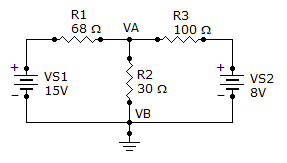# Electrical Engineering - Branch, Loop and Node Analyses - Discussion

Discussion Forum : Branch, Loop and Node Analyses - General Questions (Q.No. 2)
2.
What is the current through R2?3.19 A
319 mA
1.73 A
173 mA
Explanation:
No answer description is available. Let's discuss.
Discussion:
16 comments Page 2 of 2.

Applying kvl to the circuit,

((v-15)/68)+(v/30)+((v-8)/100).
On solving above expression we get 5.171v.

Current at R2= 5.17/30=0.172 amps.
172 milli amps.

Ashish singhal said:   1 decade ago
By appling mesh analysis

15=68i'+ 30(i'-i")
15=98i'-30i"

Now at second battery

-8=100i"+30*(i'-i")
-8=-30i'+130i"

After calculation

i'=.145
i"= -.028

So total current in 30 ohm resistance

i' - i"

0.145 - (-0.028)

= 0.173 A or

= 173 mA.

Rita J said:   1 decade ago
Applying kcl at node A and taking node B as refrence:

(15-va)/68-va/30-(va-8)/100=0

va=5.1689v;

Ir2=va/30=5.1689/30=0.172A=172mA;